Ex 3.6

Chapter 3 Class 10 Pair of Linear Equations in Two Variables (Term 1)
Serial order wise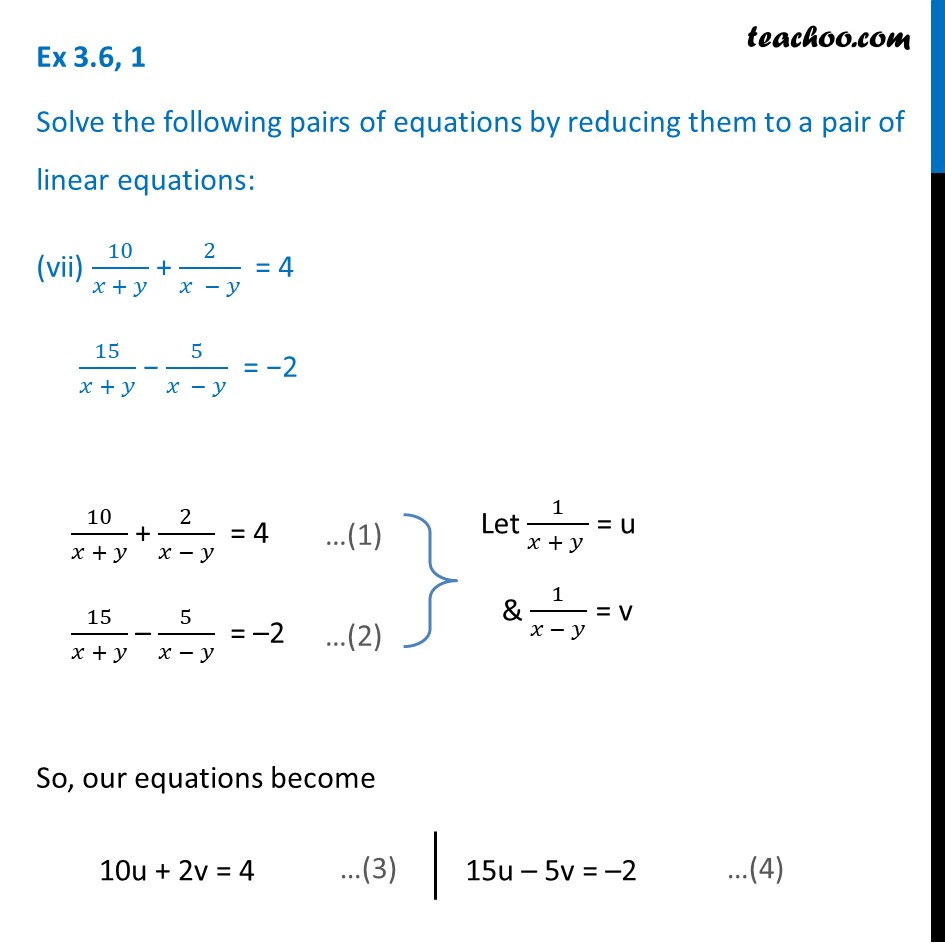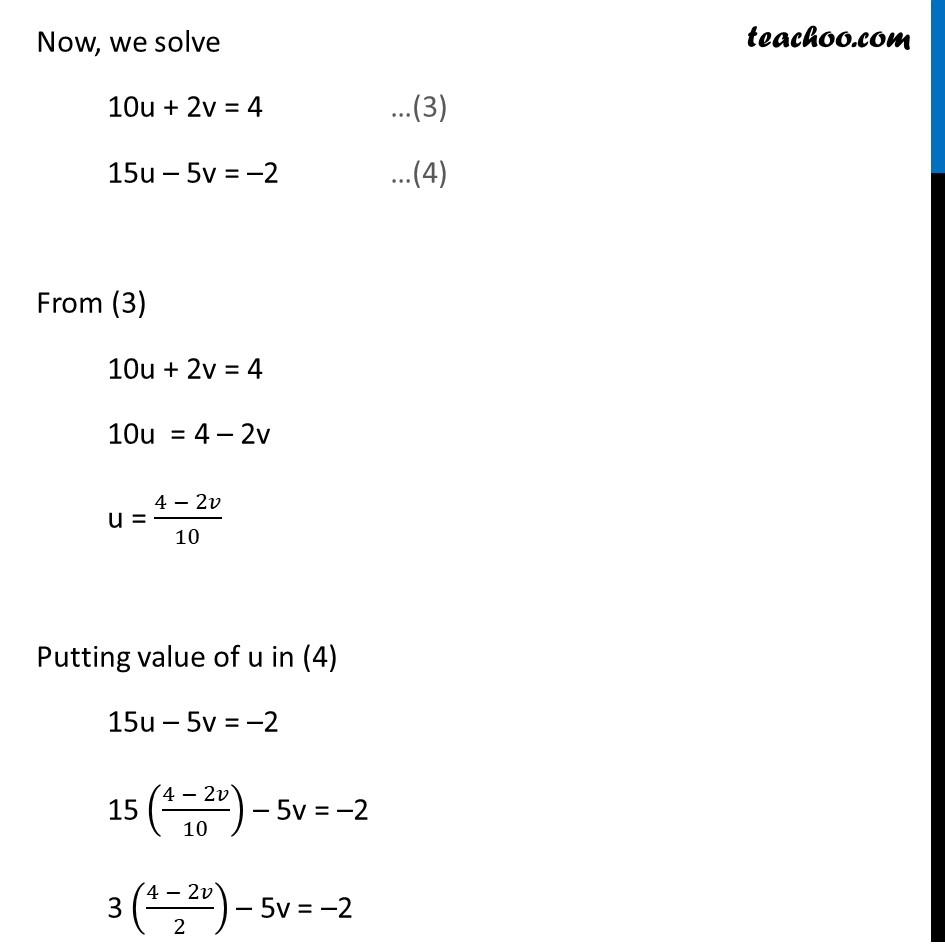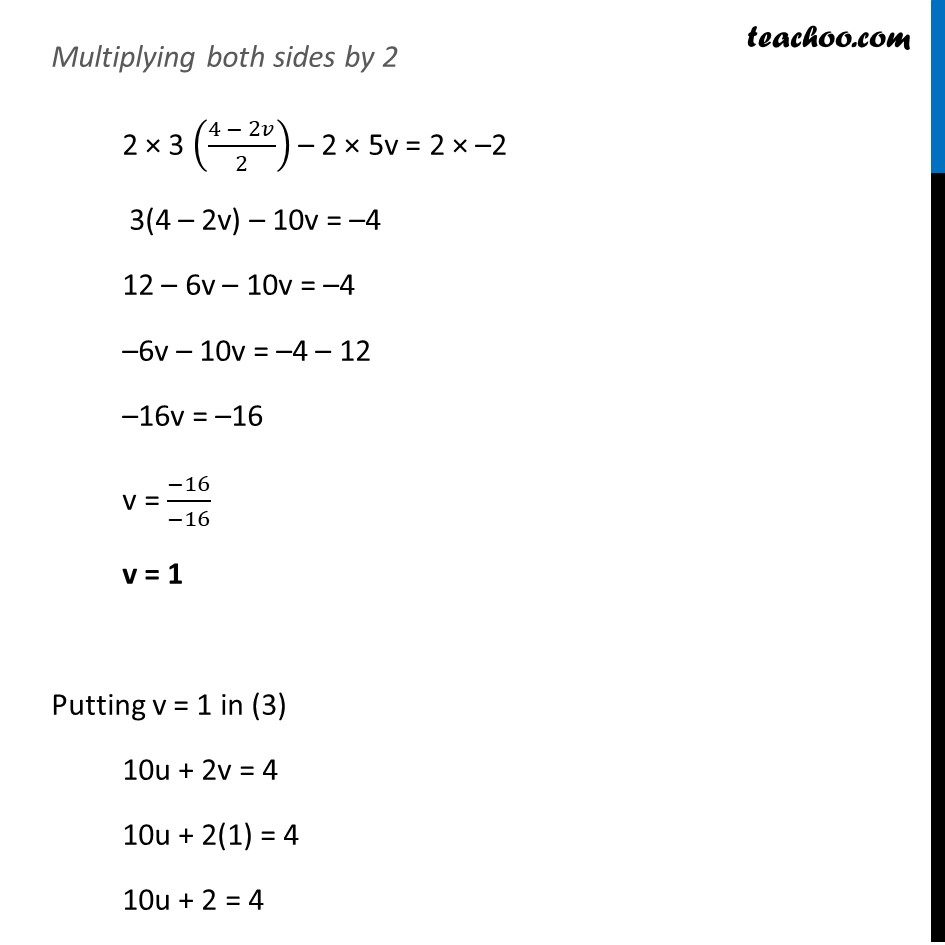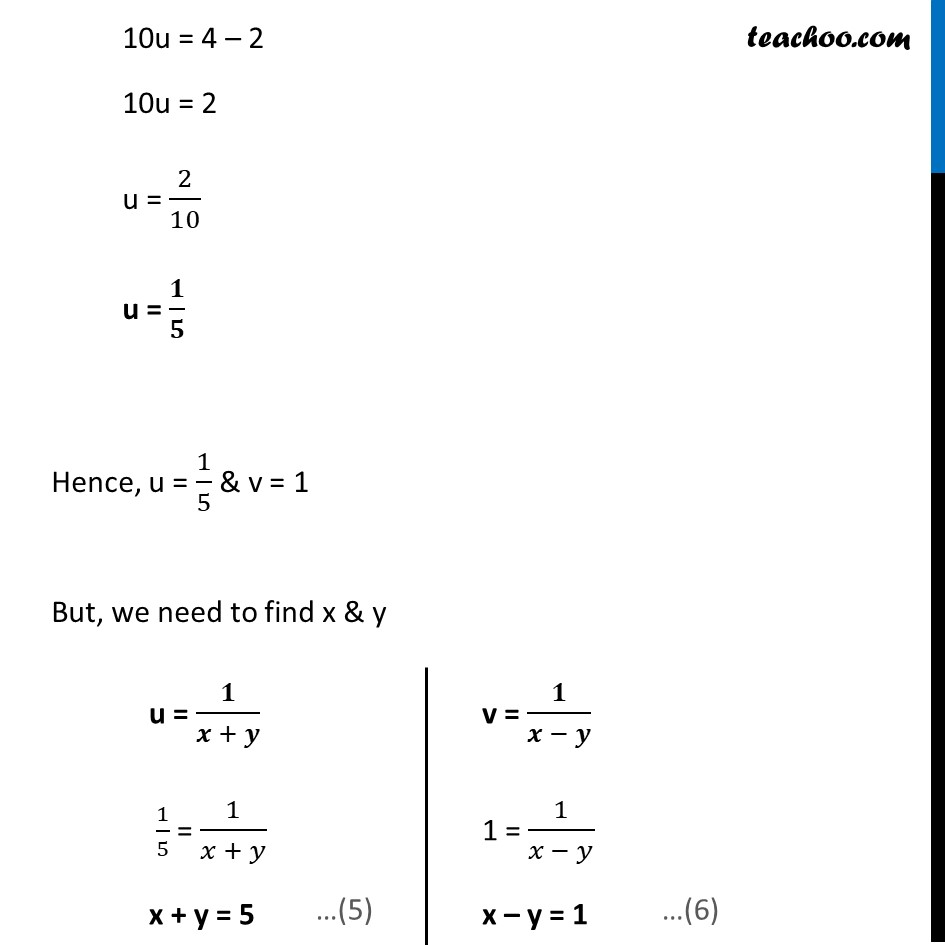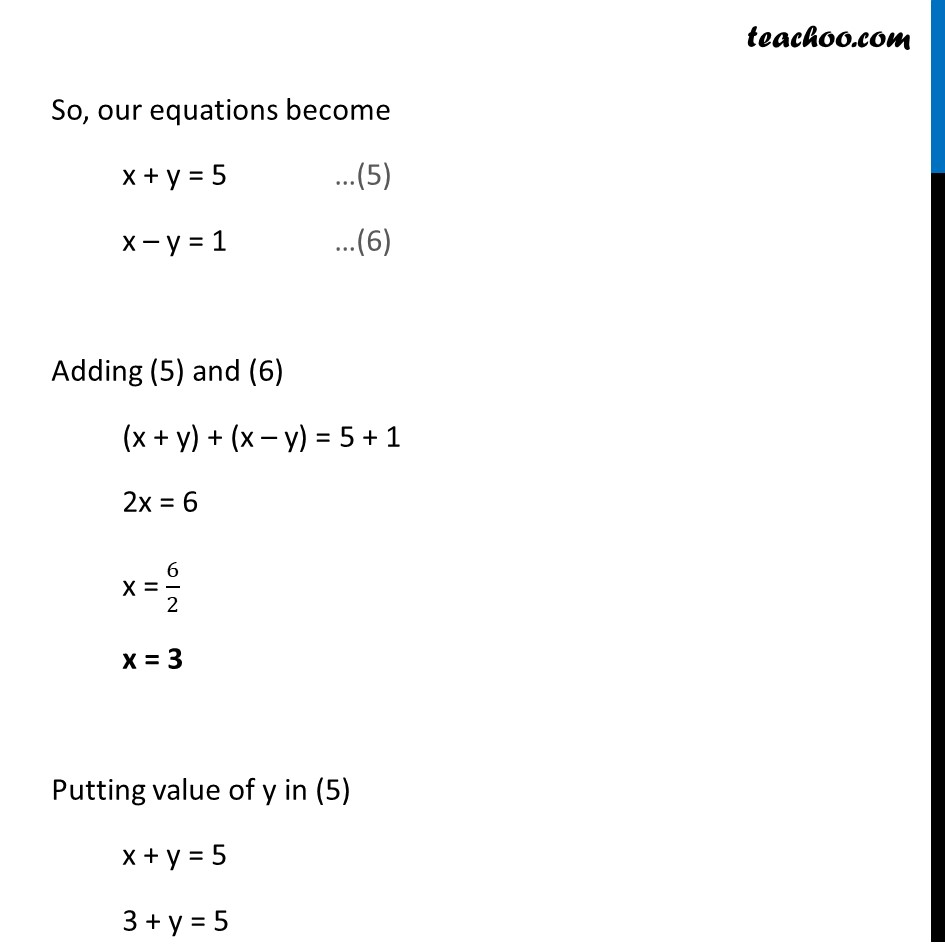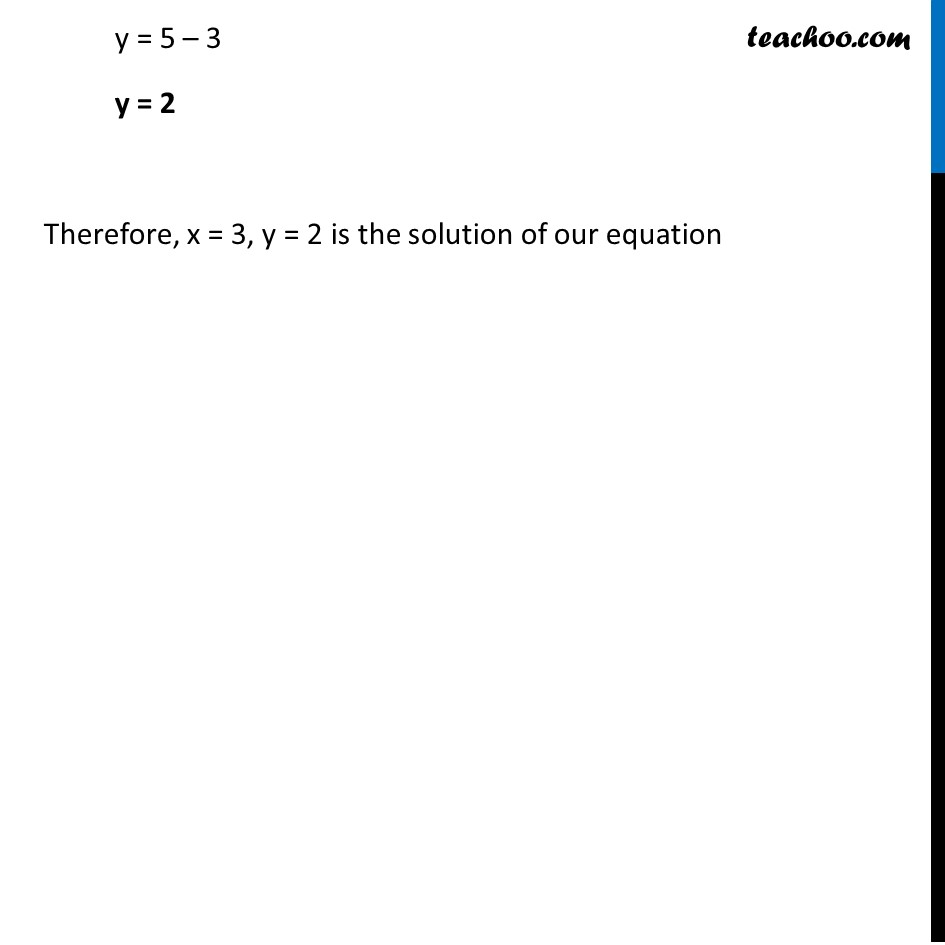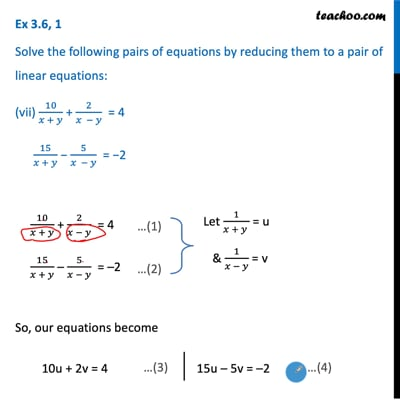This video is only available for Teachoo black users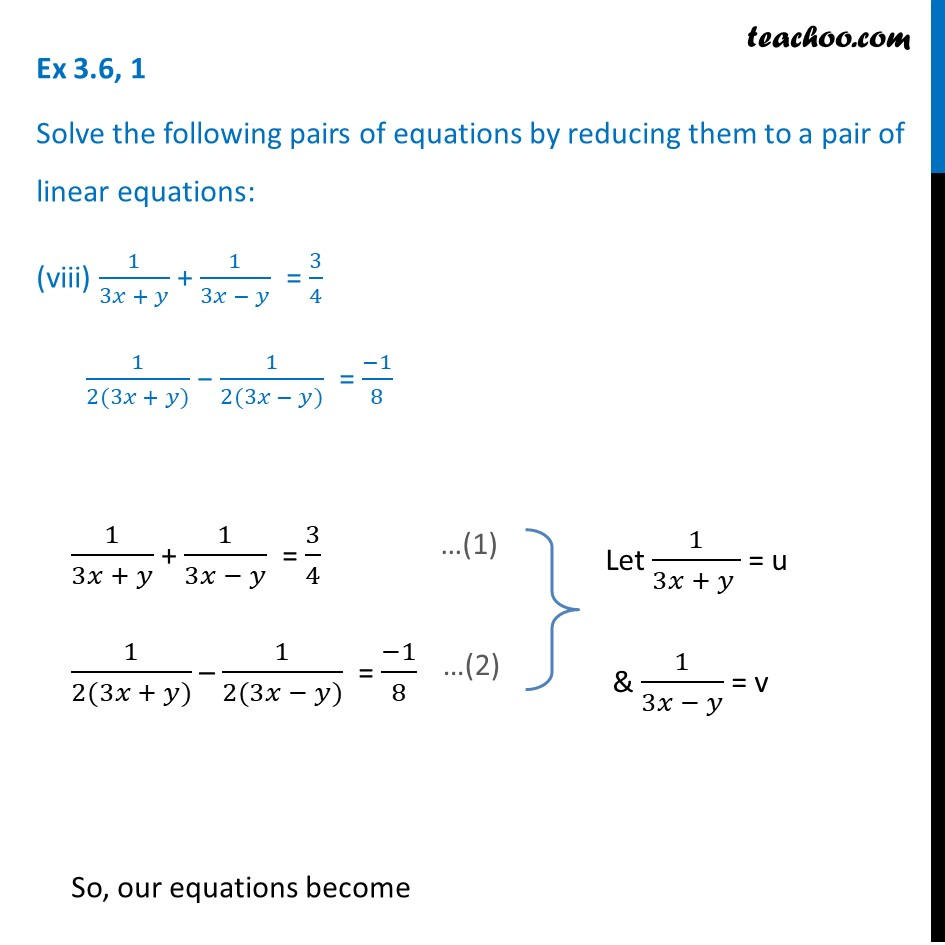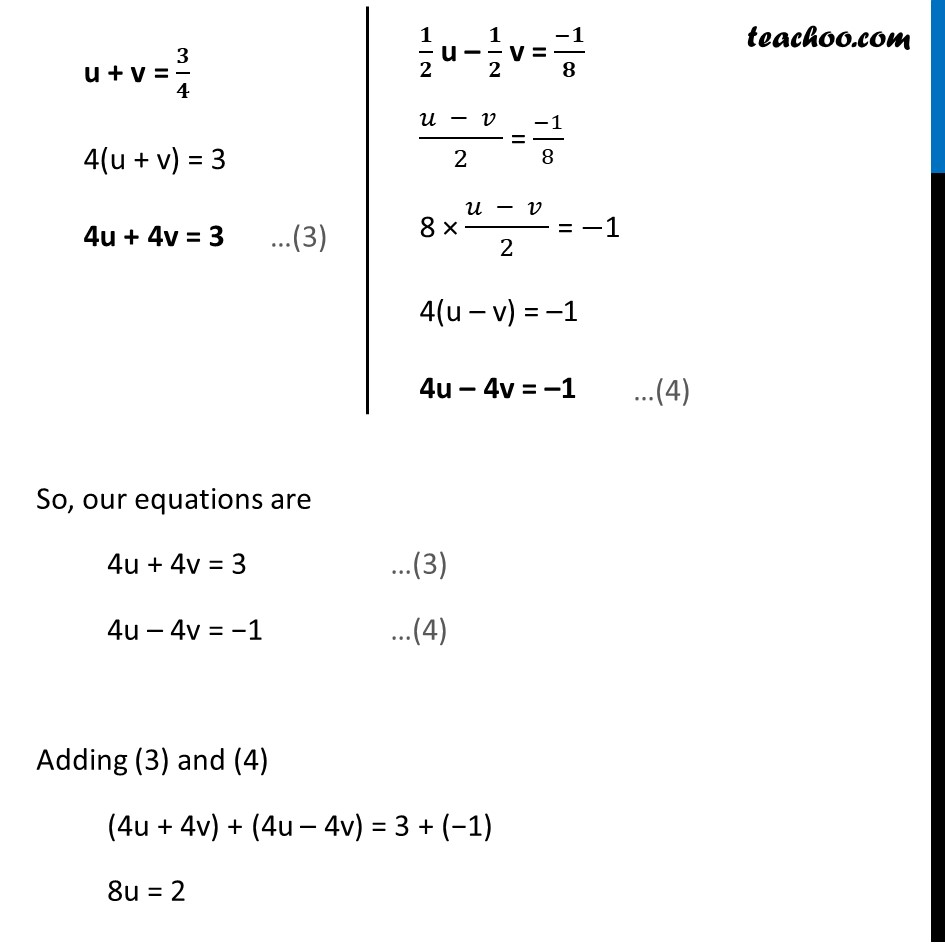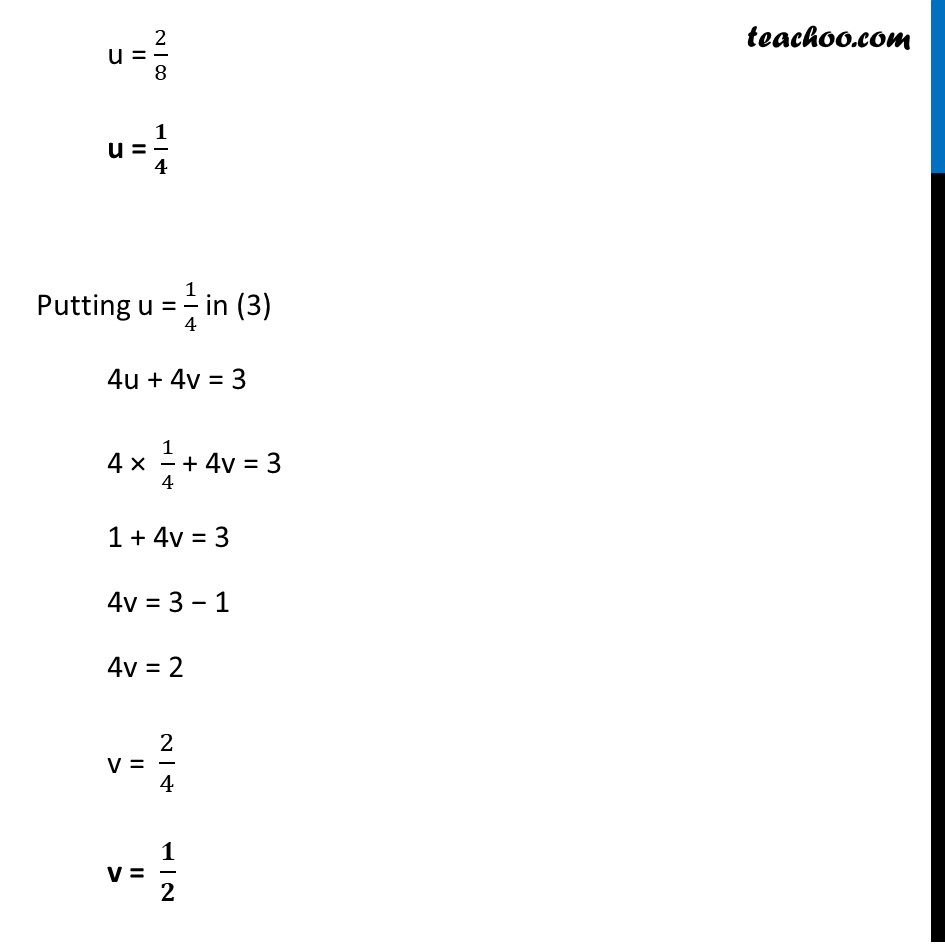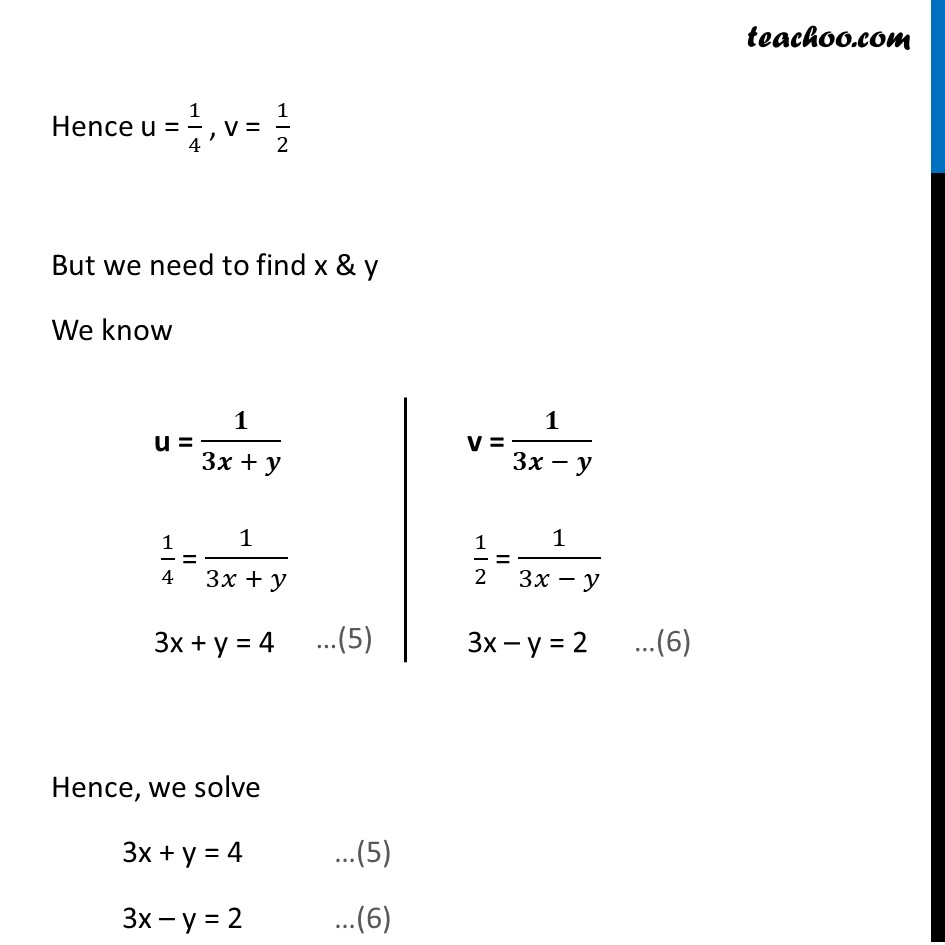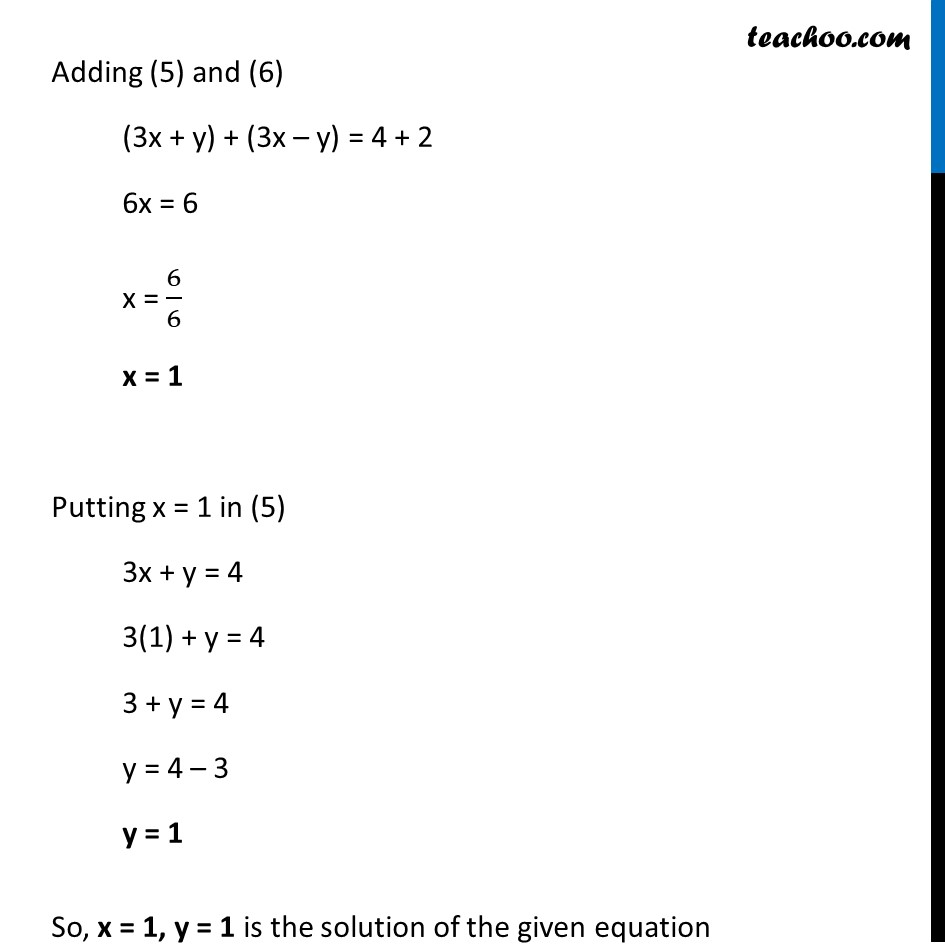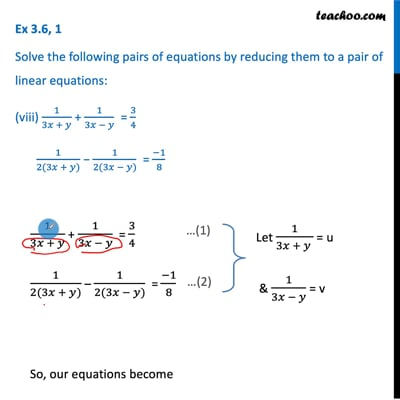This video is only available for Teachoo black users

### Transcript

Ex 3.6, 1 Solve the following pairs of equations by reducing them to a pair of linear equations: (vii) 10/(𝑥 + 𝑦) + 2/(𝑥 − 𝑦) = 4 15/(𝑥 + 𝑦) − 5/(𝑥 − 𝑦) = −2 10/(𝑥 + 𝑦) + 2/(𝑥 − 𝑦) = 4 15/(𝑥 + 𝑦) – 5/(𝑥 − 𝑦) = –2 So, our equations become 10u + 2v = 4 15u – 5v = –2 Now, we solve 10u + 2v = 4 …(3) 15u – 5v = –2 …(4) From (3) 10u + 2v = 4 10u = 4 – 2v u = (4 − 2𝑣)/10 Putting value of u in (4) 15u – 5v = –2 15 ((4 − 2𝑣)/10) – 5v = –2 3 ((4 − 2𝑣)/2) – 5v = –2 Multiplying both sides by 2 2 × 3 ((4 − 2𝑣)/2) – 2 × 5v = 2 × –2 3(4 – 2v) – 10v = –4 12 – 6v – 10v = –4 –6v – 10v = –4 – 12 –16v = –16 v = (−16)/(−16) v = 1 Putting v = 1 in (3) 10u + 2v = 4 10u + 2(1) = 4 10u + 2 = 4 10u = 4 – 2 10u = 2 u = 2/10 u = 𝟏/𝟓 Hence, u = 1/5 & v = 1 But, we need to find x & y u = 𝟏/(𝒙 + 𝒚) 1/5 = 1/(𝑥 + 𝑦) x + y = 5 v = 𝟏/(𝒙 − 𝒚) 1 = 1/(𝑥 − 𝑦) x – y = 1 So, our equations become x + y = 5 …(5) x – y = 1 …(6) Adding (5) and (6) (x + y) + (x – y) = 5 + 1 2x = 6 x = 6/2 x = 3 Putting value of y in (5) x + y = 5 3 + y = 5 y = 5 – 3 y = 2 Therefore, x = 3, y = 2 is the solution of our equation Ex 3.6, 1 Solve the following pairs of equations by reducing them to a pair of linear equations: (viii) 1/(3𝑥 + 𝑦) + 1/(3𝑥 − 𝑦) = 3/4 1/(2(3𝑥 + 𝑦)) − 1/(2(3𝑥 − 𝑦)) = (−1)/8 1/(3𝑥 + 𝑦) + 1/(3𝑥 − 𝑦) = 3/4 1/(2(3𝑥 + 𝑦)) – 1/(2(3𝑥 − 𝑦)) = (−1)/8 So, our equations become u + v = 𝟑/𝟒 4(u + v) = 3 4u + 4v = 3 𝟏/𝟐 u – 𝟏/𝟐 v = (−𝟏)/𝟖 (𝑢 − 𝑣 )/2 = (−1)/8 8 × (𝑢 − 𝑣 )/2 = −1 4(u – v) = –1 4u – 4v = –1 So, our equations are 4u + 4v = 3 …(3) 4u – 4v = −1 …(4) Adding (3) and (4) (4u + 4v) + (4u – 4v) = 3 + (−1) 8u = 2 u = 2/8 u = 𝟏/𝟒 Putting u = 1/4 in (3) 4u + 4v = 3 4 × 1/4 + 4v = 3 1 + 4v = 3 4v = 3 − 1 4v = 2 v = 2/4 v = 𝟏/𝟐 Hence u = 1/4 , v = 1/2 But we need to find x & y We know u = 𝟏/(𝟑𝒙 + 𝒚) 1/4 = 1/(3𝑥 + 𝑦) 3x + y = 4 v = 𝟏/(𝟑𝒙 − 𝒚) 1/2 = 1/(3𝑥 − 𝑦) 3x – y = 2 Hence, we solve 3x + y = 4 …(5) 3x – y = 2 …(6) Adding (5) and (6) (3x + y) + (3x – y) = 4 + 2 6x = 6 x = 6/6 x = 1 Putting x = 1 in (5) 3x + y = 4 3(1) + y = 4 3 + y = 4 y = 4 – 3 y = 1 So, x = 1, y = 1 is the solution of the given equation